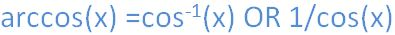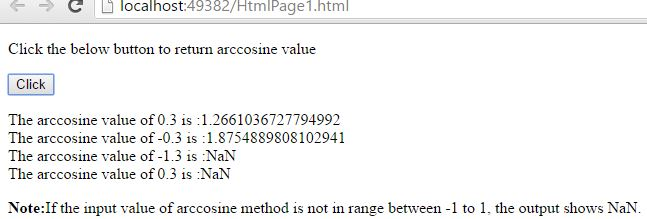# arccosine (acos()) in JavaScript

##### How to return the arccosine of a number in JavaScript?

The `acos()` method is nothing but the arccosine function in mathematics. Arccosine function is the inverse function of cosine function. This function returns the arccosine in radians of a number, and the returned value is between 0 and π radians. In mathematics shortform of arccosine  is 'arccos'.In JavaScript, by using `acos()` method we can return the arccosine of a number.

NOTE: The input value of `acos()` method must be in between the -1 to 1, to return the correct arccosine of a number. If the input number is outside the range -1 to 1, the output returns 'NaN'.

### Returning the arccosine of a number in between the range 1 to -1

```<p>Click the below button to return arccosine value</p>
<input type="button" onclick="myNumber()" value="Click">
<p id="myId"></p>

<script>
function myNumber(){
document.getElementById("myId").innerHTML = Math.acos(-0.3);
}
</script>
```

In the above code snippet we have given `Id` as "`myId`"to the second `<p>` element in the HTML code. There is a function myNumber() in the`<script>`block which is connected to the onclick of the HTML button. We need to return the arccosine of a number, for that we are using `acos()` method. Onclick of the button "Click" in the HTML code fires the function myNumber() in the `<script>` block at the same time `Math.acos()` method returns the arccosine value of a given input number as output.

OUTPUT### Returning the arccosine of different values

```<p>Click the below button to return arccosine value</p>
<input type="button" onclick="myFunction()" value="Click">
<p id="myId"></p>

<script>
function myFunction() {
var a = Math.acos(0.3);
var b = Math.acos(-0.3);
var c = Math.acos(-1.3);
var d = Math.acos(1.3);

var r = "The arccosine value of 0.3 is :" + a
+"<br>" + "The arccosine value of -0.3 is :" + b
+ "<br>" + "The arccosine value of -1.3 is :" + c
+ "<br>" +"The arccosine value of 0.3 is :" + d;
document.getElementById("myId").innerHTML = r;
}
</script>

<p><strong>Note:</strong>If the input value of arccosine method is not in range between -1 to 1, the output shows NaN.</p>
```

Arccosine of different values

Onclick of the button "Click" in the HTML code fires the function myFunction() in the `<script>` block at the same time `Math.acos()` method returns the arccosine values of a given input numbers as output.

OUTPUT### Returning the arccosine value of the number enetered by the user

```<input type="button" onclick="myNumber()" value="Click to Enter Vlue">

<script>
function myNumber() {
val = prompt("Enter the value in between -1 to +1 to know the exact Arccosine value:", "");
alert('Arccosine value of entered number is::'+Math.acos(val));
}
</script>
```

Arccosine value of a number enter by user

In the above code snippet there is a function myNumber() in the `<script>` block which is connected to the onclick of the HTML button. We need to return the arccosine value of a number which is entered by the user, for that we are using `Math.acos(val)` method in the alert box. Onclick of the button in the HTML code fires the function in the `<script>` block at same time user asked to enter the value in the input box, after entering the value and then clicking on 'OK' button gives the arccosine value of a entered number as output in the alert box.

OUTPUTIn the above output after clicking the 'Click to Enter Value' button, the user asked to enter the input value. The user entered the input value '0.3' in the above output, after entering the input value click on 'OK' button to know the arccosine value of entered number.Views: 4087 | Post Order: 96